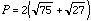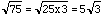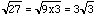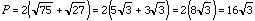Date: Sun, 24 Jan 1999 04:19:48 -0600 (CST) Subject: radicals Name: Randy Who is asking: Student Level: All Question: Find the perimeter of a rectangle in simplified radical form L=root80 W=root45. I would appreciate a detailed explination of the process for solving. Thanks Randy Hi Randy Here is a similar problem that is not the same as your homework. Find the perimeter of a rectangle with L=root75 and W=root27. Since the figure is a rectangle its perimeter is 2(L + W) which is. Radicals behave like algebraic terms and fractions and you can only add like terms so you can't directly add the radicals in this expression. The secret here is to see 75 as the product of a perfect square and another factor, that is 75 = 25 x 3. Thus. Likewise. ThusCheers Jack and Penny Go to Math Central To return to the previous page use your browser's back button.